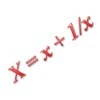#### You may also like### Curve Fitter

Can you fit a cubic equation to this graph?### The Why and How of Substitution

Step back and reflect! This article reviews techniques such as substitution and change of coordinates which enable us to exploit underlying structures to crack problems.

# Polynomial Interpolation

##### Age 16 to 18 Challenge Level:

Steve wishes to draw a quadratic polynomial $y(x) = ax^2+bx+c$ through the three points $(x, y)=(1,4), (2, 5), (3, 7)$. He writes down this expression:

$$y(x) = 4\frac{(x-2)(x-3)}{(1-2)(1-3)}+5\frac{(x-1)(x-3)}{(2-1)(2-3)}+7\frac{(x-1)(x-2)}{(3-1)(3-2)}$$

Does this solve the problem that Steve was trying to address?

Using Steve's example as a guide, can you construct a quadratic polynomial which passes through the three points $(1,2), (2, 4), (4, -1)$? Is this the only such quadratic polynomial?

Can you construct a cubic polynomial which passes through the four points $(1,2), (2, 4), (3, 7),(4, -1)$. Is this the only such cubic polynomial?

By this stage, the particular examples you have constructed should give you ideas about how to construct a 'general' case. Use your insights to answer these questions:

Can you write down an expression for a line passing through the two points $(2, 7)$ and $(8,-6)$ using this method?

Can you always fit a quadratic polynomial through three points $(x_1, y_1), (x_2, y_2), (x_3, y_3)$?

Can you always fit a quartic polynomial through five points $(x_i, y_i)$ ($i=1\dots 5$) where exactly two of $x_i$ are zero?

How many different polynomials can you construct which would pass through the points $(1, 1), (2, 2), (3, 3), (4, 4), (5, 5)$?

Explore sets of points through which it is not possible to fit a polynomial.

Extension: For various numbers of points and degrees of polynomial you might wish to consider when the fitting is unique, when it is possible with multiple polynomials and when it is impossible.